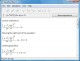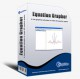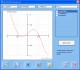# equation 3 0 reviews and downloads

Search results for «equation 3 0»:

Showing 1 to 10 from 10 matchesEquation Wizard 1.21 Automatically solves algebraic equations of any order written in any form SharewarePDF Equation 0.7 PDF Equation gives you quick access to LaTeX's typesetting power in almost every Mac OS X application. FreewareEquation Service 1.0 Equation Service is a program that uses pdflatex to produce small PDF files containing equations and other text. FreewareChemical Equation Expert 2.12a Chemical Equation Expert is an integrated tool for chemistry professionals FreewareEquation Grapher 2.2 Equation grapher is a free graphing calculator to graph equations. FreewareDeadLine 2.36 Analyze, solve, and plot various mathematical equations. FreewareQuotient 1.4 Have you ever wondered what happens to the quotient of a division equation that goes off the screen of your calculator, such as 1/7? How long is it? Does it repeat? It does indeed repeat. Freeware3D Grapher 1.21 Plot and animate 2D and 3D equation and table-based graphs with ease SharewareODEcalc 6.1 ODEcalc (tm): Ordinary Differential Equation (ODE) Calculator! Solves ODEs FreewarePhysics Cheat Sheet 1.2 Physics Cheat Sheet is an interactive physics package that helps students solve and visualize numerous physics equations. Demo